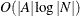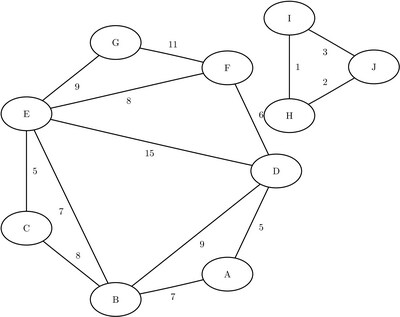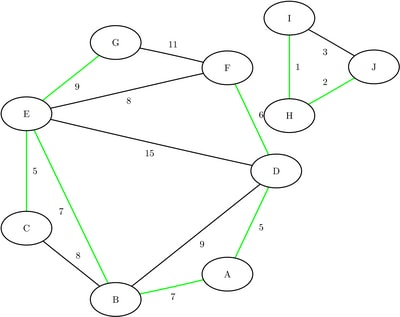### Minimum Spanning Tree

A spanning tree of a connected undirected graph is a subgraph that is a tree that connects all the nodes together. Given weights on the links, a minimum spanning tree (MST) is a spanning tree whose weight is less than or equal to the weight of every other spanning tree. More generally, any undirected graph (not necessarily connected) has a minimum spanning forest, which is a union of minimum spanning trees of its connected components.

In PROC OPTNET, the minimum spanning tree algorithm can be invoked by using the MINSPANTREE statement. The options for this statement are described in the section MINSPANTREE Statement. This algorithm can be used only on undirected graphs.

The resulting minimum spanning tree is given in the output data set that is specified in the OUT= option in the MINSPANTREE statement.

PROC OPTNET uses Kruskal’s algorithm (Kruskal 1956) to compute the minimum spanning tree. This algorithm runs in timeand therefore should scale to very large graphs.

#### Minimum Spanning Tree for a Simple Undirected Graph

As a simple example, consider the weighted undirected graph in Figure 2.39.

Figure 2.39: A Simple Undirected GraphThe links data set can be represented as follows:

```data LinkSetIn;
input from \$ to \$ weight @@;
datalines;
A B  7  A D  5  B C 8  B D 9  B E 7
C E  5  D E 15  D F 6  E F 8  E G 9
F G 11  H I  1  I J 3  H J 2
;
```

The following statements calculate a minimum spanning forest and output the results in the data set `MinSpanForest`:

```proc optnet
minspantree
out     = MinSpanForest;
run;
```

The data set `MinSpanForest` now contains the links that belong to a minimum spanning forest, which is shown in Figure 2.40.

Figure 2.40: Minimum Spanning Forest

from to weight
H I 1
H J 2
C E 5
A D 5
D F 6
B E 7
A B 7
E G 9
42

The minimal cost links are shown in green in Figure 2.41.

Figure 2.41: Minimum Spanning ForestFor a more detailed example, see Example 2.5.